# Solution assignment 18 Quadratic equations (factorizing)

### Assignment 18

Try to solve by factorizing: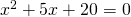### Solution

We have to find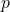and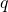such that: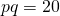and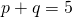There are no whole numbersenthat satisfy these equations. This does not mean that there are no solutions. In this case the equation has no solutions because the discriminant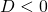, see also Quadratic equations (abc-formula).

0